## 连续优化与组合优化

### 组合优化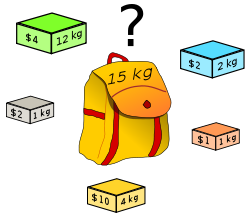### 元启发式方法(Metaheuristic)

a metaheuristic is a higher-level procedure or heuristic designed to find, generate, or select a heuristic (partial search algorithm) that may provide a sufficiently good solution to an optimization problem

• 遗传算法(Genetic Algorithms，GA)
• 模拟退火(Simulated Annealing，SA)
• 蚁群算法(Ant Colony Optimization，ACO)
• 粒子群算法(Particle Swarm Optimization，PSO)
……

Metaheuristics in Combinatorial Optimization: Overview and Conceptual Comparison

## 遗传算法

Genetic algorithms are commonly used to generate high-quality solutions to optimization and search problems by relying on bio-inspired operators such as mutation, crossover and selection。

### 基本概念

• 个体（individual） : 一个候选解
• 种群（generation） : 一组候选解，代表某一代的所有个体
• 染色体（chromosomes） : 候选解的染色体表示，常见的用01字符串表示
• 适应度（fitness） : 个体的适应度，一般与优化问题中的目标函数值相关
• 选择（selection）: 根据个体适应性从一个 generation 中选出 适应性好的进入下一代
• 交叉（crossover） : 类似于生物中两个个体进行杂交，两个 chromosomes 进行相应的交叉
• 变异（mutation）: chromosomes 的某一位进行突变，如从0变到1或从1变到0

1. 可行解的表示方法，也就是常说的编码方式，上面说到了常用的有 01 编码，除此之外，根据问题的不同，还存在其他的一些编码方式
2. 适应度函数，也就是计算上面适应度的函数，这个函数一般yong就是用目标函数，如要最大化某个函数，则将这个函数作为目标函数即可，反之最小化的时候加个负号即可。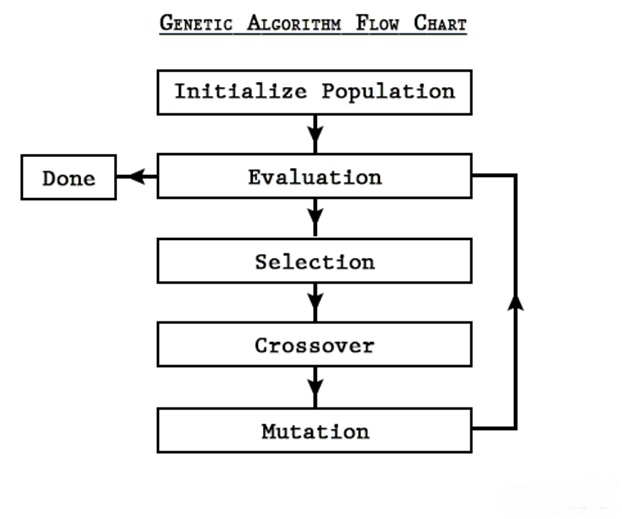### 算法流程

#### 选择(Selection)

$$p_i = \frac{f_i}{\sum_{j=1}^{M} f_j}$$

#### 交叉(crossover)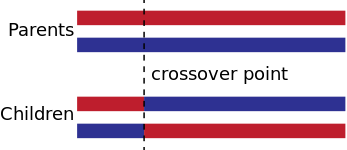#### 变异(mutation)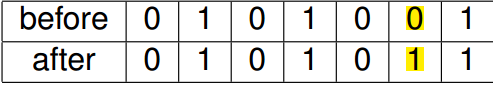#### 终止(termination)

• A solution is found that satisfies minimum criteria
• Fixed number of generations reached
• Allocated budget (computation time/money) reached
• The highest ranking solution’s fitness is reaching or has reached a plateau such that successive iterations no longer produce better results
• Manual inspection
• Combinations of the above

### 例子

#### 遗传算法解决背包问题

$$max \sum_{i=1}^{15} x_iv_i\ s.t. \sum_{i}w_ix_i \le W\ x_i \in \lbrace 0,1 \rbrace$$

$x_i \in \lbrace 0,1 \rbrace$ 表示是否将第 $i$ 件物品放到背包中。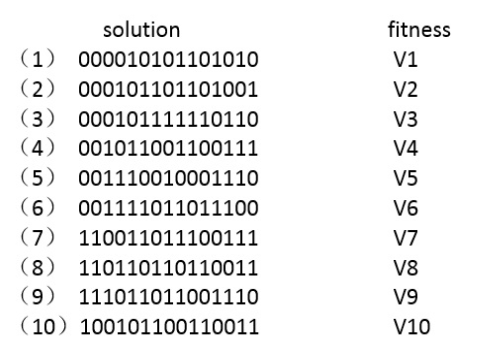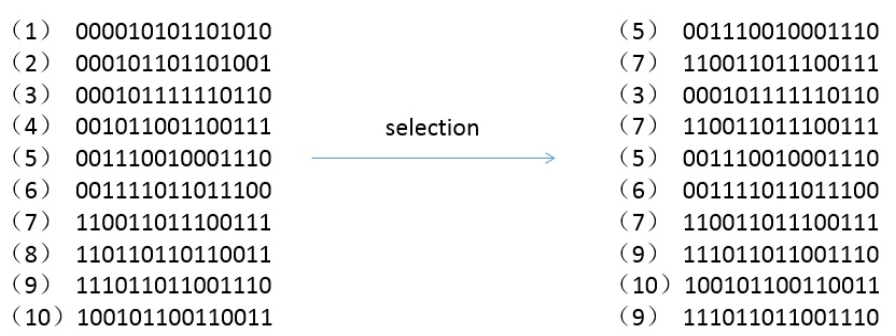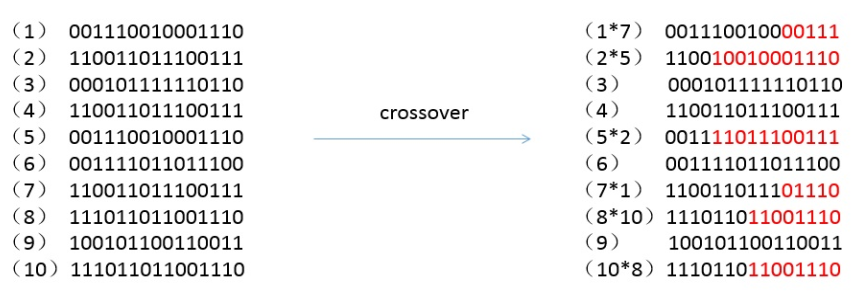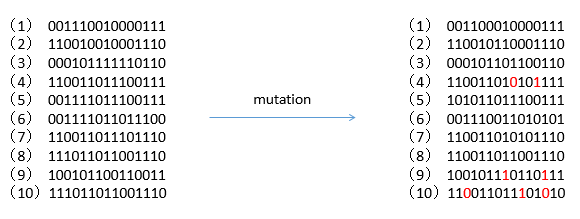#### 遗传算法解决连续优化问题

$$\max f(x) = x + 10sin(5x) + 7cos(4x)， x \in [0,9]$$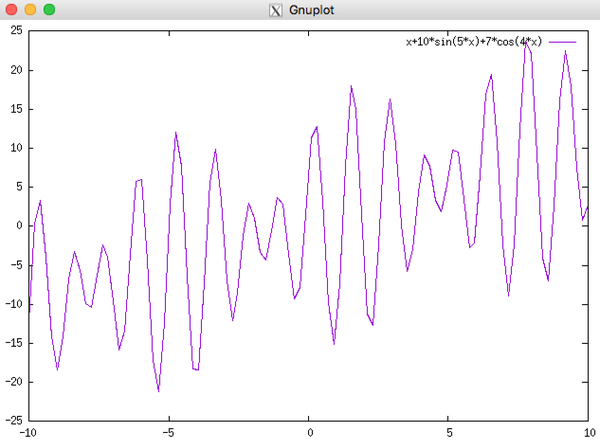x = 0 + decimal(chromosome)×(9-0)/(2^17-1)

decimal( ): 将二进制数转化为十进制数

lower_bound: 函数定义域的下限
upper_bound: 函数定义域的上限
chromosome_size: 染色体的长度通过上述公式，我们就可以成功地将二进制染色体串解码成[0,9]区间中的十进制实数解。

## 理论基础

### 模式定理(Schema Theory)

#### 选择

$$m(s, t+1) = M\frac{m(s,t) \overline {f(s)}}{\sum_{j=1}^{M} f_j} = m(s,t) \frac{\overline {f(s)}}{\overline {f}}$$

$$m(s, t+1) = m(s,t) \frac{\overline {f(s)}}{\overline {f}} = m(s,t) \frac{(1+c)\overline f }{\overline {f}} = m(s,t)(1+c)$$

$$m(s, t+1) = m(s, t)(1+c)^t$$

#### 交叉

$$m(s, t+1) = m(s,t) \frac{\overline {f(s)}}{\overline {f}}[1 - p_c \frac{\delta(s)}{\lambda - 1}]$$

#### 变异

$$m(s, t+1) = m(s,t) \frac{\overline {f(s)}}{\overline {f}}[1 - p_c \frac{\delta(s)}{\lambda - 1} - p_mo(s)]$$

### 收敛性分析

1. 传统的遗传算法不能收敛到全局最优
2. 在传统的遗传算法基础上每次选择的时候保留前面所有迭代里面的最优个体，最终能收敛到全局最优。

## 改进方案(Variants)

### 编码方式

• 便于提高遗传算法的局部搜索能力
• 交叉、变异等遗传操作便于实现
• 符合最小字符集编码原则
• 便于利用模式定理对算法进行理论分析

### 精英选择

Removing the Genetics from the Standard Genetic Algorithm 对其做了详细的描述。

### 参数自适应

$$P_c= \begin{cases} \frac{k_1(f_{max}-f’)}{f_{max}-f_{avg}}&& {f’ \geq f_{avg}}\\ k_2 && {f’ < f_{avg}} \end{cases}$$

$$P_m= \begin{cases} \frac{k_3(f_{max}-f’)}{f_{max}-f_{avg}} && {f’ \geq f_{avg}}\\ k_4 && {f’ < f_{avg}} \end{cases}$$

$$P_c= \begin{cases} p_{cmin}-\frac{（p_{cmax}-p_{cmin}）(f’-f_{avg})}{f_{max}-f_{avg}}&& {f’ \geq f_{avg}}\\ p_{cmax} && {f’ < f_{avg}} \end{cases}$$

$$P_m= \begin{cases} p_{mmin}-\frac{（p_{mmax}-p_{mmin}）(f’-f_{avg})}{f_{max}-f_{avg}}&& {f’ \geq f_{avg}}\\ p_{mmax} && {f’ < f_{avg}} \end{cases}$$

$p_{cmin}$, $p_{cmax}$ 分别表示交叉率取值的下限和上限；而$p_{mmin}$, $p_{mmax}$ 分别表示变异率取值的下限和上限,这样就避免了前面的概率出现0的情况。

## 参考文献

Blum C, Roli A. Metaheuristics in combinatorial optimization: Overview and conceptual comparison[J]. ACM Computing Surveys (CSUR), 2003, 35(3): 268-308.

Chu P C, Beasley J E. A genetic algorithm for the multidimensional knapsack problem[J]. Journal of heuristics, 1998, 4(1): 63-86.

Goldberg, David .Genetic Algorithms in Search, Optimization and Machine Learning[M].MA: Addison-Wesley Professional, 1989:ISBN 978-0201157673

Rudolph G. Convergence analysis of canonical genetic algorithms[J]. IEEE transactions on neural networks, 1994, 5(1): 96-101

Srinivas M, Patnaik L M. Adaptive probabilities of crossover and mutation in genetic algorithms[J]. IEEE Transactions on Systems, Man, and Cybernetics, 1994, 24(4): 656-667.

Dandy G C, Simpson A R, Murphy L J. An improved genetic algorithm for pipe network optimization[J]. Water resources research, 1996, 32(2): 449-458.

Baluja S, Caruana R. Removing the genetics from the standard genetic algorithm[C].Machine Learning: Proceedings of the Twelfth International Conference. 1995: 38-46.

Srinivas M, Patnaik L M. Adaptive probabilities of crossover and mutation in genetic algorithms[J]. IEEE Transactions on Systems, Man, and Cybernetics, 1994, 24(4): 656-667.